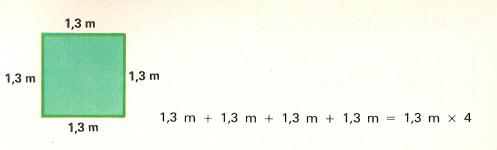5. Multiplication of decimalsEach side of a square is 1.3 meters. How much is the sum of its four sides? We can add or subtract.

1,3
x    4
--------
5,2

When a whole number is multiplied by 10, we can simply add a zero to the end. Example: 65 x 10 = 650

When a whole number is multiplied by 100, we add two zeros to the end: Example: 7 x 100 = 700

When a whole number is multiplied by 1000, we add three zeros to the end. Example: 523 x 1000 = 523000

Solve these multiplications:

 5 x 100  = 48 x 10 = 7 x 1000 = 32 x 10 = 128 x 100 =

To multiply a decimal by 10, 100, 1000 and so on, we have to move the decimal point to the right as many places as there are zeros in the factors. If it is necessary, we add extra zeros.

Example: 5,4 x 10 = 54;  0,7 x 100 = 70;  0,123 x 1000 = 123

 0,01 x 100  = 15,3 x 1000 = 0,00025 x 10 = 2,1 x 100 = 0,25 x 1000 =

To multiply a decimal number by a whole number, first we have to ignore the decimal point and multiply the digits. Then we have to place the decimal point in the result so that it has the same number of decimal places as the number being multiplied

Ejemplo:    2,57
x    6
--------
15,42

Escribe estas operaciones sobre un papel y resuelve las operaciones.

 127 x 9,5  = 6,25 x 5 = 1,26 x 17 = 9,20 x 15 = 147 x 12,1 =

To multiply a decimal by another decimal, we must ignore the decimal points and multiply the digits. Then, we must count the total number of decimal places in both decimal numbers being multiplied; and we must place a decimal point in the result so that it has the same number of decimal places as the total number of decimal places in the two numbers being multiplied.

Ejemplo:     13,5
x   0,03
--------
0,405

Write these operations on a paper and solve these problems.

 5,3 x 1,12  = 3,4 x 6,12 = 1,15 x 12,25 = 1,125 x 300,7 = 8,32 x 1,01 =

Ask how formulate problemas and then solve them:

 A father gave 2.55 euros to each of his three children. How much money did he give to all of them? One bottle of wine has 1.50 liters and another bottle has 2.50 liters. How much liters does the two bottles have in total? One bread is 0.75 kilograms. How much will seven breads weigh? Santiago had \$ 8.75 and he spent \$ 3.25 on school supplies. How much money does he have? A pear is 0.12 kilos. How much will 9 pears weigh?

 | Educational applications | Mathematics | In Spanish  | To print

®Arturo Ramo García.-Record of intellectual property of Teruel (Spain) No 141, of 29-IX-1999
Plaza Playa de Aro, 3, 1ş DO 44002-TERUEL